# 目标检测系列(3)—又一个 one stage 的目标检测模型—SSD(下)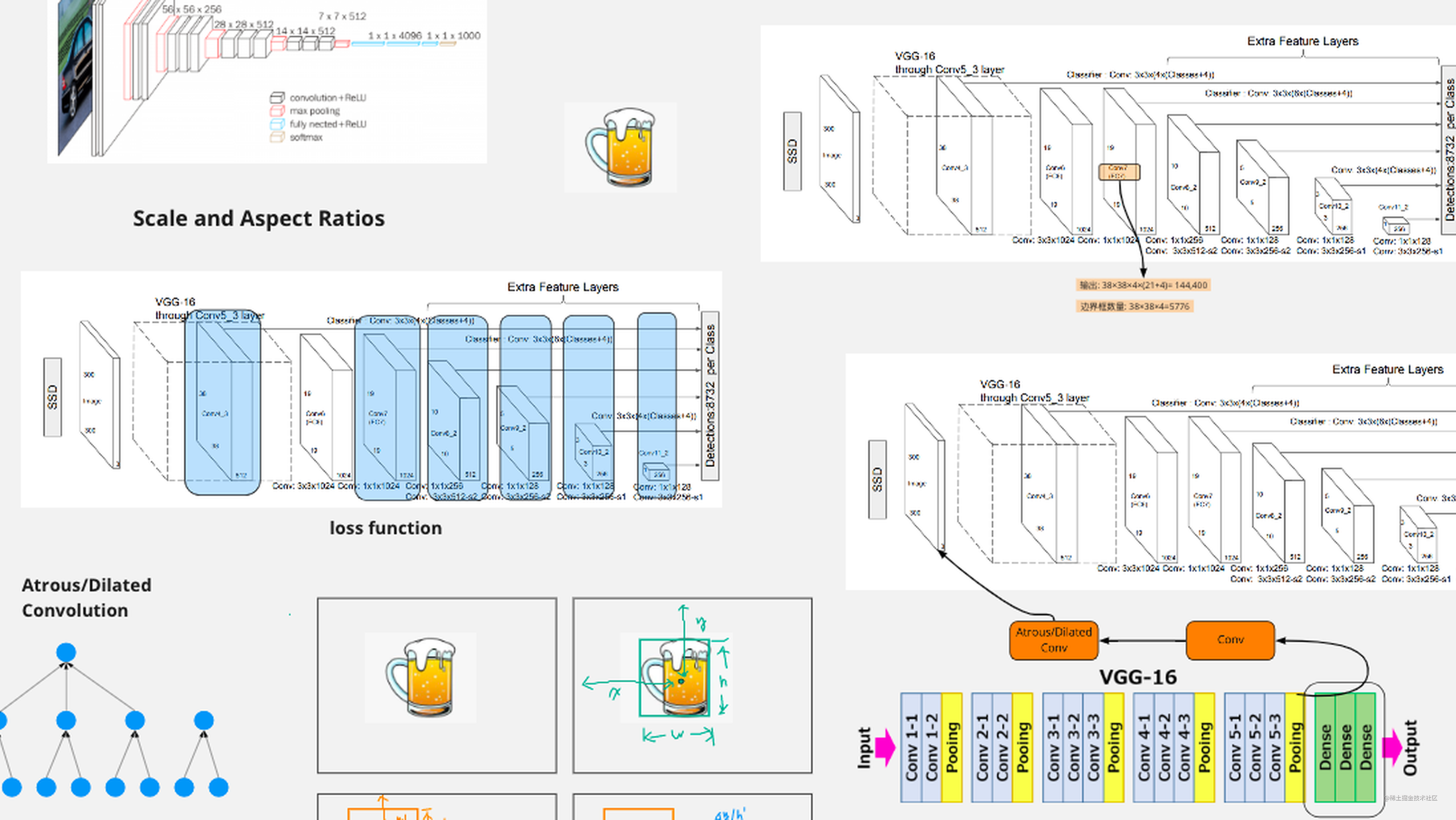### 位置损失函数

$t_x^j = (g_x^j - p_x^i)/p_w^i\\ t_y^j = (g_y^j - p_y^i)/p_h^i\\ t_w^j = \log(g_w^j/p_w^i)\\ t_h^j = \log(g_h^j/p_h^i)$

### 默认框(Default box)的缩放尺度和宽高比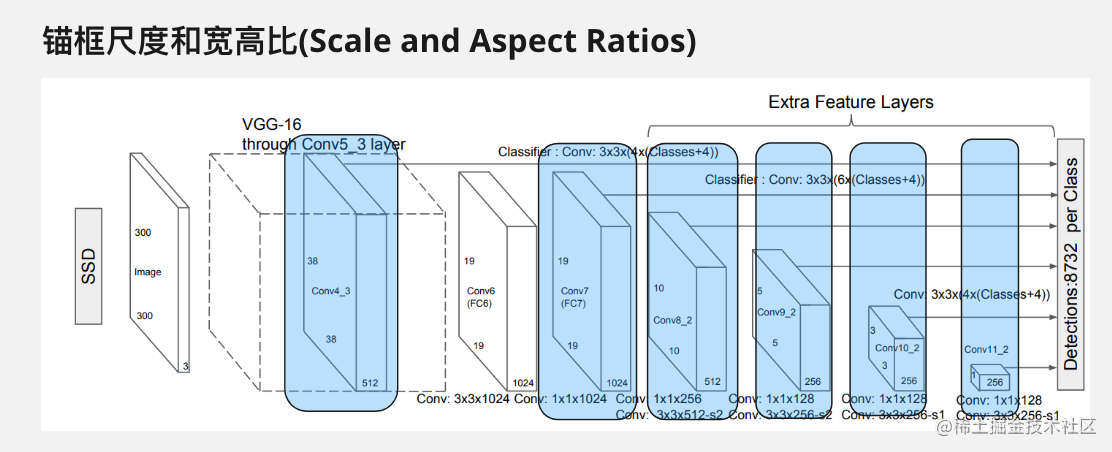$(w_k^a,h_k^a) = (s_k\sqrt{a_r},s_k/\sqrt{a_r})$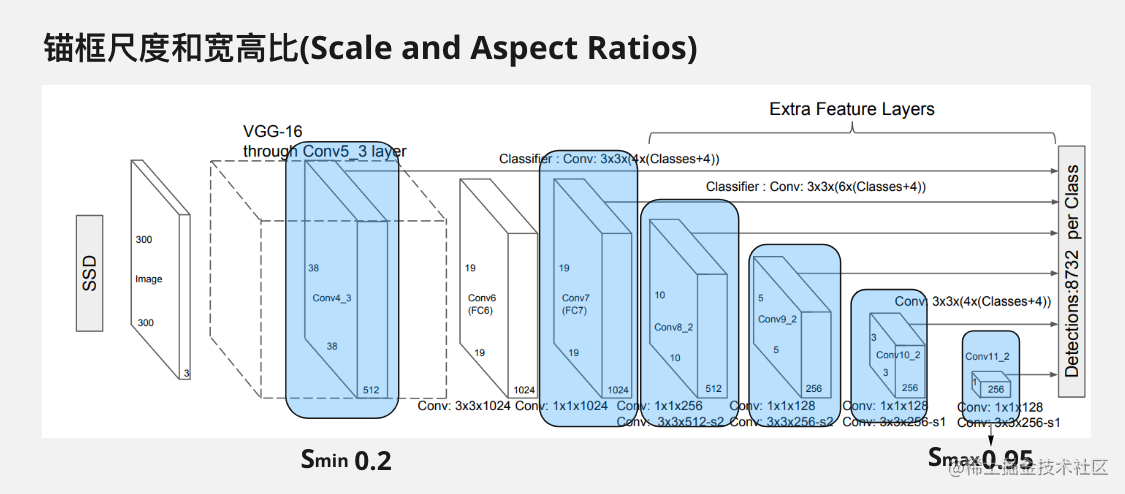##### 计算 $S_k$ 的公式
$S_k = S_{min} + \frac{S_{max} - S_{min}}{m - 1}(k-1)$

• $k$ 表示第 k 特征层，那么 k 取值范围也就是 1 到 m，当 k 取 1 时，$S_k$ = $S_{min}$，当 $k$ 取 m 时候 $S_k$ 就等于 $S_{max}$ 这一点不难看出
• 假设 $S_{min} = 0.2$,$S_{max} = 0.95$$m=6$ 条件下，那么公式就等于
$S_k = 0.2 + \frac{0.95 - 0.2}{6-1}(k-1) = 0.2 + 0.15(1-k)$
##### $a_r$

$a_r \in \{1,2,3,\frac{1}{2},\frac{1}{3}\}$
##### 举一个例子

$S_4 = 0.2 + 0.15(4-1) = 0.65\\ w_4^a = 0.65 \sqrt{1/2} = 0.46\\ h_4^a = 0.65 / \sqrt{1/2} = 0.92$

$S_k^{\prime} = \sqrt{S_kS_{k+1}}$
$S_{5} = 0.2 + 0.15(1-5) = 0.8 \\ S_4 = \sqrt{S_4S_5} = \sqrt{0.65\times 0.8} =0.72$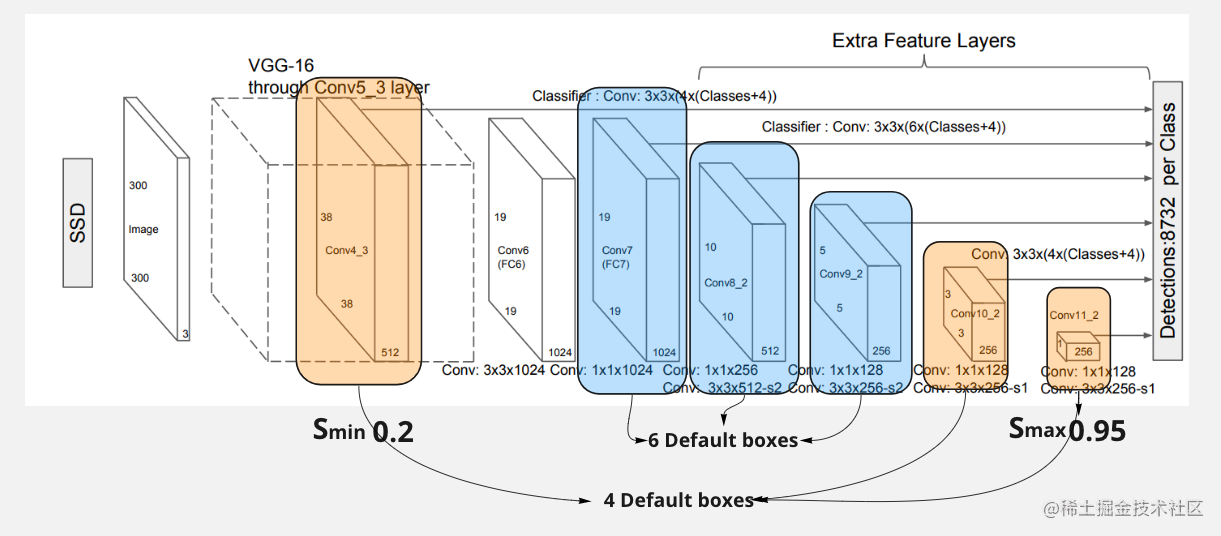#### 对于默认框中心点的取值

$(c_x,c_y) = \left( \frac{i+0.5}{|f_k|},\frac{j+0.5}{|f_k|} \right)$

1$38 \times 38$211,2,1/2$38 \times 38 \times 4$
2$19 \times 19$451,2,3,1/2,1/3$19 \times 19 \times 6$
3$10 \times 10$991,2,3,1/2,1/3$10 \times 10 \times 6$
4$5 \times 5$1531,2,3,1/2,1/3$5 \times 5 \times 6$
5$3 \times 3$2071,2,1/2$3 \times 3 \times 4$
6$1 \times 1$2611,2,1/2$1 \times 1 \times 4$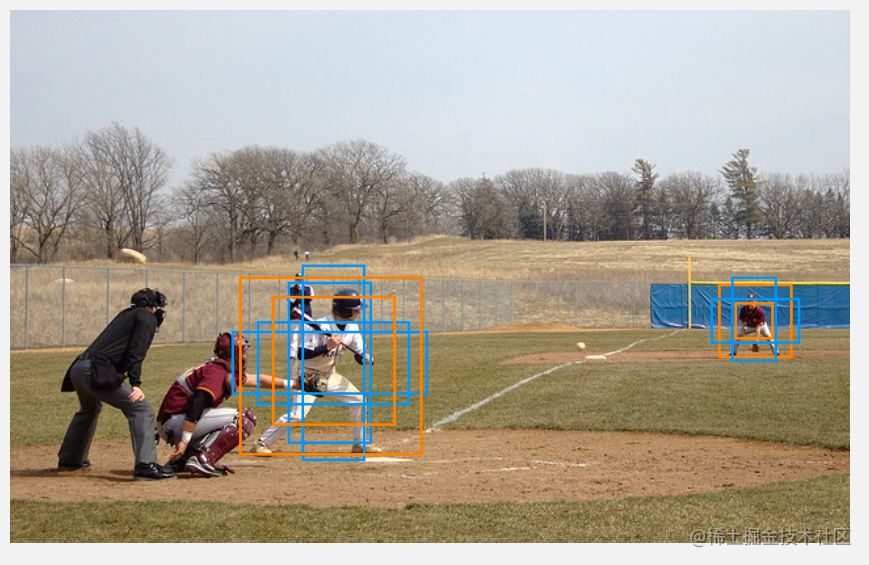• 较远处目标，有 1 个特征图负责预测，也就是会给出 4 个默认框(default boxes)分别是 1,2,1/2 还有在不比例下计算的比例为 1 的边界框
• 较近处的目标，可能由 4 特征图负责预测，那么就是给出 6 默认框

### 正负样本不均衡

• 正样本: 对于正样本选择，只要满足以下两个条件之一即可，默认框(default box)与真实框(ground truth)的 IoU 最大的默认框，还有就是对于默认框只要满足与任意真实框的 IoU 大于 0.5
• 负样本: 其实不满足正样本的默认框都应该计算为负样本，之前我们已经计算过了一共生成了 8700 多个默认框，所以还需要在这么多负样本中筛选，通过 high confidence loss 进行排序，这里 confidence loss 越大也就是网络越容易将其预测为正样本的概率越大(也就是默认框在背景处却给出很高存在目标的分数)，然后按照排序从负样本中选取数量，选取的负样本数量和正样本数量保持 3:1 的比例。

### 参考文献

• SSD: Single Shot MultiBox Detector